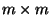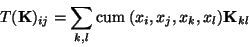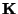Next: Weighted covariance matrix Up: Multi-unit contrast functions Previous: Non-linear PCA criteria

### Higher-order cumulant tensors

A principle of ICA estimation that is less directly connected with the objective function framework, is the eigenmatrix decomposition of higher-order cumulant tensors (defined in Appendix A). Most solutions use the fourth-order cumulant tensor, whose properties and relation to the estimation of ICA have been studied extensively [20,21,22,29,27,36]. Related methods were also introduced in . The fourth-order cumulant tensor can be defined as the following linear operator T from the space ofmatrices to itself:(21)

where the subscript ij means the (i,j)-th element of a matrix, andis amatrix. This is a linear operator, and thus has m2 eigenvalues that correspond to eigenmatrices. Solving for the eigenvectors of such eigenmatrices, the ICA model can be estimated .

The advantage of this approach is that it requires no knowledge of the probability densities of the independent components. Moreover, cumulants can be used to approximate mutual information [36,1], as shown above, though the approximation is often very crude. The main drawback of this approach seems to be that the statistical properties of estimators based on cumulants are not very good, see Section 4.4.2.Next: Weighted covariance matrix Up: Multi-unit contrast functions Previous: Non-linear PCA criteria
Aapo Hyvarinen
1999-04-23